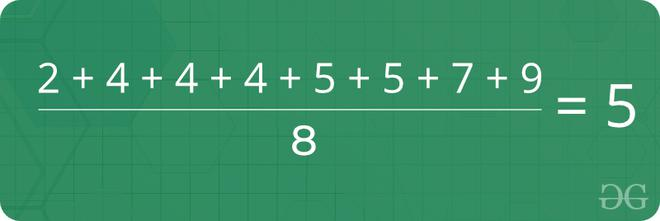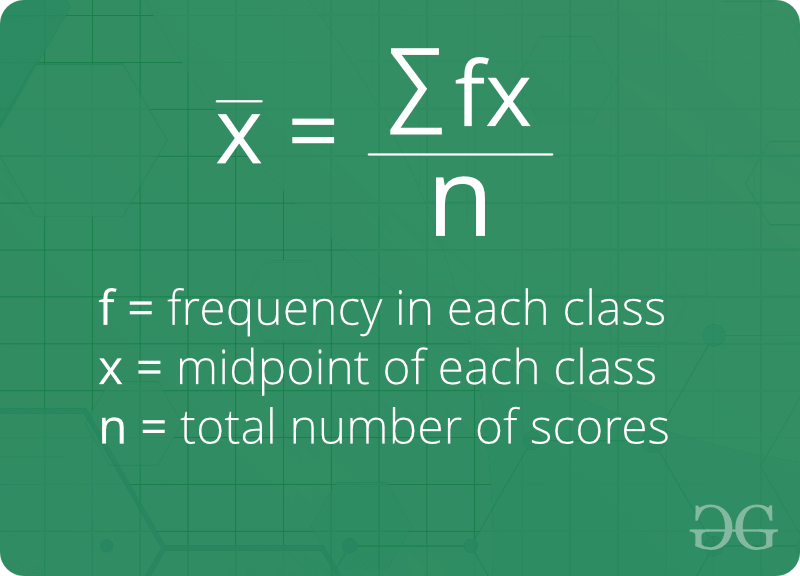GeeksforGeeks App
Open AppBrowser
Continue

# What is Mean in Statistics (Formula, Calculation, Examples & Properties)

## What is a Mean?

Mean is the average of the given numbers which is calculated by dividing the sum of given numbers by the total count of numbers

Example:

Find the mean of the given numbers 2, 4, 4, 4, 5, 5, 7, and 9?Mean of 2, 4, 4, 4, 5, 5, 7, 9

Solution:

• Step1: Take sum of all numbers, 2 + 4 + 4 + 4 + 5 + 5 + 7 + 9 = 40
• Step2: Divided sum by the total count of numbers, 40/ 8 = 5

## Properties of Mean:

1. The mean (or average) is the most popular and well-known measure of central tendency.
2. It can be used with both discrete and continuous data, although its use is most often with continuous data.
3. There are other types of means. Geometric mean, Harmonic mean, and Arithmetic mean.
4. Mean is the only measure of central tendency where the sum of the deviations of each value from the mean is always zero.

## How to find Mean of grouped data:## How to find Mean in an array?

Given the n size array, find its mean.
Examples:

Input  : {1, 3, 4, 2, 6, 5, 8, 7}
Output : Mean = 4.5
Explanation: Sum of the elements is 1 + 3 + 4 + 2 + 6 + 5 + 8 + 7 = 36, Mean = 36/8 = 4.5

Input  : {4, 4, 4, 4, 4}
Output : Mean = 4

Approach:

Formula used:

Mean of an array = (sum of all elements) / (number of elements)

Follow the steps below for implementation:

• Iterate over the array and keep adding elements to a variable sum
• Divide the sum by the size of given array.

Below is the implementation of the above approach

## C++

 `// CPP program to find mean``#include ``using` `namespace` `std;` `// Function for calculating mean``double` `findMean(``int` `a[], ``int` `n)``{``    ``int` `sum = 0;``    ``for` `(``int` `i = 0; i < n; i++)``        ``sum += a[i];` `    ``return` `(``double``)sum / (``double``)n;``}` `// Driver program``int` `main()``{``    ``int` `a[] = { 1, 3, 4, 2, 7, 5, 8, 6 };``    ``int` `n = ``sizeof``(a) / ``sizeof``(a);``    ``cout << ``"Mean = "` `<< findMean(a, n) << endl;``    ``return` `0;``}`

## Java

 `// Java program to find mean``import` `java.util.*;` `class` `GFG {` `    ``// Function for calculating mean``    ``public` `static` `double` `findMean(``int` `a[], ``int` `n)``    ``{``        ``int` `sum = ``0``;``        ``for` `(``int` `i = ``0``; i < n; i++)``            ``sum += a[i];` `        ``return` `(``double``)sum / (``double``)n;``    ``}` `    ``// Driver program``    ``public` `static` `void` `main(String args[])``    ``{``        ``int` `a[] = { ``1``, ``3``, ``4``, ``2``, ``7``, ``5``, ``8``, ``6` `};``        ``int` `n = a.length;``        ``System.out.println(``"Mean = "` `+ findMean(a, n));``    ``}``}`

## Python3

 `# Python3 program to find mean` `# Function for calculating mean`  `def` `findMean(a, n):` `    ``sum` `=` `0``    ``for` `i ``in` `range``(``0``, n):``        ``sum` `+``=` `a[i]` `    ``return` `float``(``sum` `/` `n)`  `# Driver program``a ``=` `[``1``, ``3``, ``4``, ``2``, ``7``, ``5``, ``8``, ``6``]``n ``=` `len``(a)``print``(``"Mean ="``, findMean(a, n))`

## C#

 `// C# program to find mean``using` `System;` `class` `GFG {``    ``// Function for``    ``// calculating mean``    ``public` `static` `double` `findMean(``int``[] a, ``int` `n)``    ``{``        ``int` `sum = 0;``        ``for` `(``int` `i = 0; i < n; i++)``            ``sum += a[i];` `        ``return` `(``double``)sum / (``double``)n;``    ``}` `    ``// Driver Code``    ``public` `static` `void` `Main()``    ``{``        ``int``[] a = { 1, 3, 4, 2, 7, 5, 8, 6 };``        ``int` `n = a.Length;``        ``Console.Write(``"Mean = "` `+ findMean(a, n) + ``"\n"``);``    ``}``}`

## PHP

 ``

## Javascript

 `// JavaScript program to find mean` `// Function for calculating mean``function` `findMean(a, n)``{``    ``let sum = 0``    ``for` `(``var` `i = 0; i < n; i++)``        ``sum += a[i]` `    ``return` `(sum / n)``}` `// Driver program``let a = [1, 3, 4, 2, 7, 5, 8, 6]``let n = a.length``console.log(``"Mean ="``, findMean(a, n))` `// This code is contributed by phasing17`

Output

`Mean = 4.5`

Time Complexity: O(n), Where n is the size of the given array.
Auxiliary Space: O(1), As no extra space is used.

Basic Program related to Mean

Recent Articles on Mean!

My Personal Notes arrow_drop_up